﻿ Determinantal Identities of Fibonacci, Fibonacci Like and Lucas Numbers

### Determinantal Identities of Fibonacci, Fibonacci Like and Lucas Numbers

Sanjay Harne, V.H. Badshah, Sapna SethiyaOPEN ACCESSPEER-REVIEWED

## Determinantal Identities of Fibonacci, Fibonacci Like and Lucas Numbers

Sanjay Harne1, V.H. Badshah2, Sapna Sethiya3,1Government College, Kannod(M.P.), India

2School of studies in Mathematics

3Vikram University Ujjain, India

### Abstract

Determinants have played a significant part in various areas in mathematics. For instance, they are quite useful in the analysis and solution of system of linear equations. There are different perspectives on the study of determinant. In this paper we present some determinant identities of Fibonacci and Lucas numbers.

• Harne, Sanjay, V.H. Badshah, and Sapna Sethiya. "Determinantal Identities of Fibonacci, Fibonacci Like and Lucas Numbers." Turkish Journal of Analysis and Number Theory 2.4 (2014): 110-112.
• Harne, S. , Badshah, V. , & Sethiya, S. (2014). Determinantal Identities of Fibonacci, Fibonacci Like and Lucas Numbers. Turkish Journal of Analysis and Number Theory, 2(4), 110-112.
• Harne, Sanjay, V.H. Badshah, and Sapna Sethiya. "Determinantal Identities of Fibonacci, Fibonacci Like and Lucas Numbers." Turkish Journal of Analysis and Number Theory 2, no. 4 (2014): 110-112.

 Import into BibTeX Import into EndNote Import into RefMan Import into RefWorks

### 1. Introduction

It is well known that the Fibonacci numbers and polynomials are of great importance in the study of many subjects such as algebra, geometry, combinatorics, approximation theory, graph theory and number theory itself.

They occur in a variety of other fields such as finance, art, architecture, music, etc.

One may notice several practical and effective instruments for calculating determinants in the nice survey articles  and . Much attention has been paid to the evaluation of determinants of matrices, especially when their entries are given recursively . There is a long tradition of using matrices and determinants to study Fibonacci numbers. Bicknell – Johnson and Spears  use elementary matrix operationand determinants to generate classes of identities for generalized Fibonacci numbers. Cahill and Narayan  show how Fibonacci and Lucas numbers arise as determinants of some tridiagonal matrices. T. Benjamin, T. Cameron and J. Quinn , provides combinatorial interpretations for Fibonacci identities using determinants. Koshy  explained two chapters on the useof matrices and determinants in Fibonacci numbers.

Spivey  describe the sum property for determinants and presented new proofs of identities like the Cassini identity, the d'Ocagne identity and the Catalan identity.

Macfarlane  use the property for determinants to give new identities involving Fibonacci and related number is defined by the recurrence relation,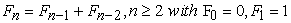Lucas sequence is defined by the recurrence relation,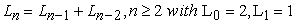The Fibonacci- Like sequence  is defined by recurrence relation,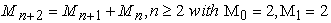first few numbers of Fibonacci-Like sequences are 2, 2, 4, 6, 10, 16, and so on

Theorem 1 For every integer n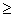0 :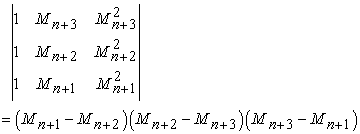Theorem 2 For every integer n0: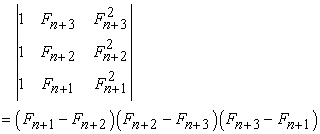Theorem 3 For every integer n0: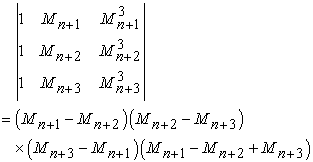Theorem 4 For every integer n0: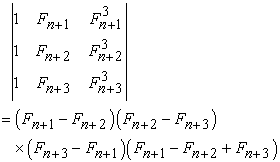Theorem 5 For every integer n0: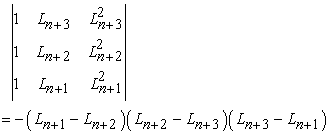Theorem 6 For every integer n0: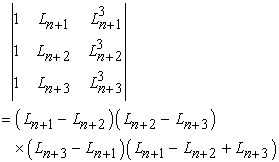Theorem 7 For every integer n0: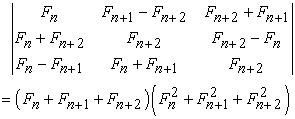Theorem 8 For every integer n0: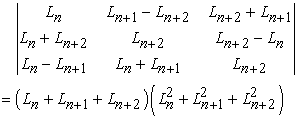Theorem 9 For every integer n0: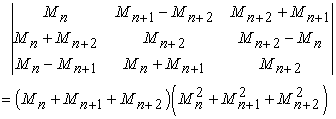Theorem 10 For every integer n0: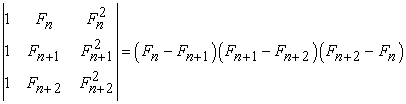Theorem 11 For every integer n0: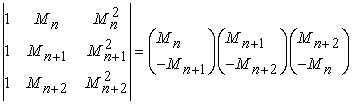Theorem 12 For every integer n0: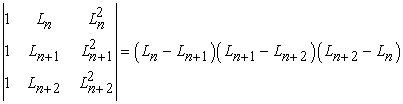Theorem 13 For every integer n0: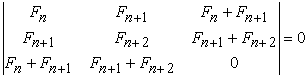Theorem 14 For every integer n0: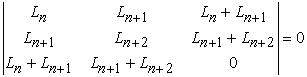Theorem 15 For every integer n0: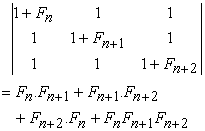Theorem 16 For every integer n0: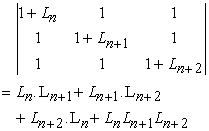Theorem 17 For every integer n0: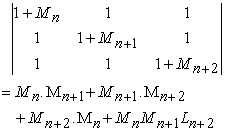Theorem 18 For every integer n0: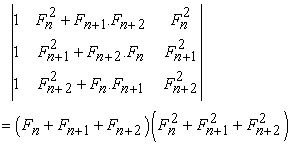### Acknowledgment

We are thankful to referees for their valuable comments.

### References

  Benjamin A., Cameron N. and Quinn J.: Fibonacci Determinants- A Combinatorial Approach, Fibonacci Quarterly, 39-55 (1), 2007, Vol 45.In article  Bicknell-Johnson M. and Spears C. P.:Classes Of Identities For the Generalized Fibonacci number Gn= Gn-1 + Gn-2, n ≥ 2. from Matrices with Constantvalued Determinants, Fibonacci Quarterly, 121-128 (2), 1996, Vol. 34.In article  B. Singh, O. Sikhwal, and S. Bhatnagar, Fibonacci-Like Sequence and its properties, Int.J. Contemp Math.Sciences, Vol.5, 2010, No.18, 857-868.In article  Cahill N. and Narayan D.:Fibonacci and Lucas numbers s Tridigonal Matrix Determinants, Fibonacci Quarterly, 216-221 (3), 2004, Vol. 42.In article  Koshy T.:Fibonacci and Lucas Numbers with Applications, Wiley, 2001.In article CrossRef  Krattenthaler C.: Advanced determinant calculus, Seminaire Lotharingien Combin, Article, b42q, 67, 1999.In article  Krattenthaler C.: Advanced determinant calculus: A Complement, Liner Algebra Appl., 68-166.In article  Macfarlane A. J.: Use of Determinants to Present Identities Involving Fiboncci and Related Numbers, Fibonacci Quarterly, 68-7648(1), 2010, Vol. 48.In article  Spivey M. Z.: Fibonacci Identities via the Determinant sum property, College Mathematics Journal, 286-289 (4), 2006, Vol. 37.In article